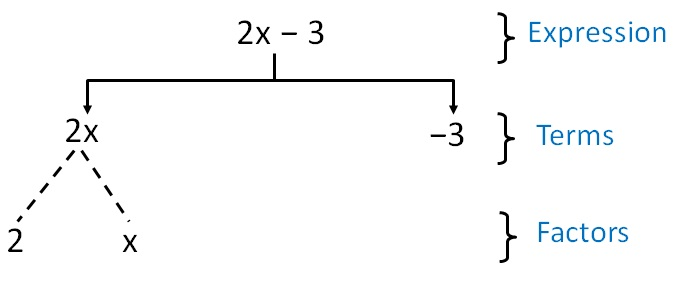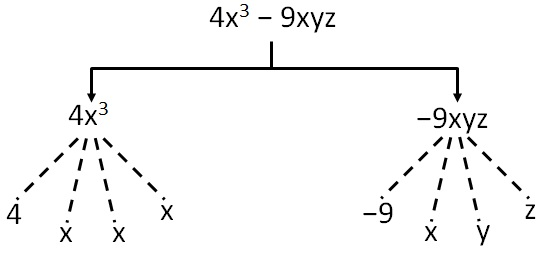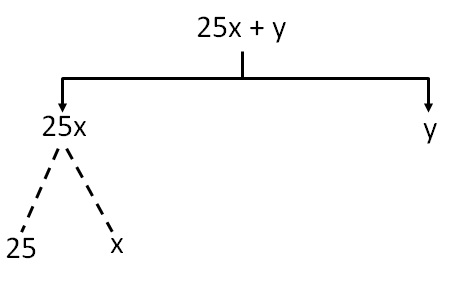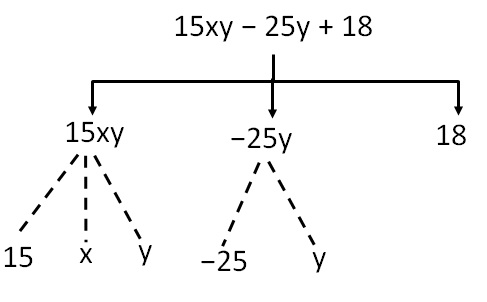Terms, Factors and Coefficients

Chapter 10 Class 7 Algebraic Expressions
Concept wise

Suppose we have an expressionHere,

The terms are 2x & −3

& factors of 2 are 2, x

Here, −3 is a constant

### For 4x 3 − 9xyzThe terms are 4x 3 and −9xyz

and factors of 4x 3 are 4, x, x and x

and factors of −9xyz are −9 , x, y, z

## 25x + yThe terms are 25x + y

and factors of 25x are 25  and x

factors of y is y

### 15xy − 25y + 18The terms are 15xy, –25y and 18

and factors of 15xy are  15, x  and y,

factors of  −25y are −25 and y

Here, 18 is a constant

The above tree formed to find terms & factors is called a tree diagram

We can also find terms & factors using table

 Expression Terms Factors 2x − 3 2x −3 2, x − 3 4x 3 − 9xyz 4x 3 −9xyz 4, x, x, x −9, x, y, z 25x + y 25x   y 25, x   y 15xy − 25y + 18 15xy −25y 18 15, x, y −25, y 18

Learn in your speed, with individual attention - Teachoo Maths 1-on-1 Class